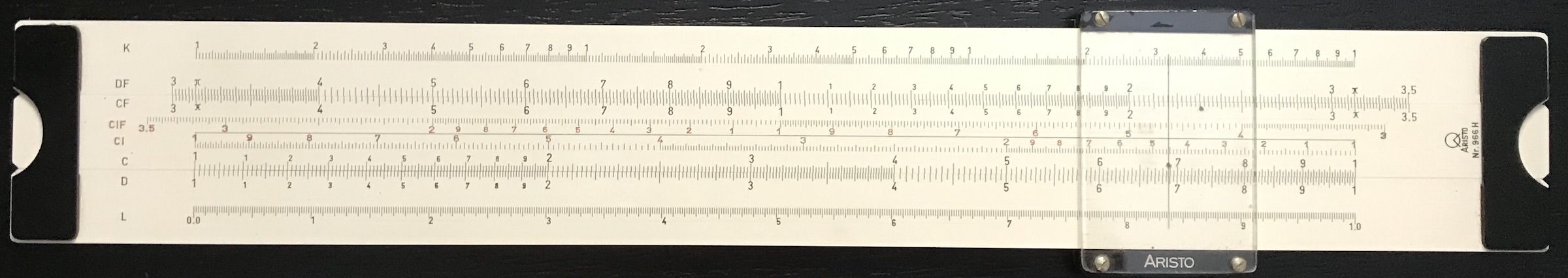## 3.6 Inverse and Folded Scales

One often finds inverse scales on a slide rule, such as CI and DI. The CI scale, for instance, is just the C scale printed in reverse. Since the logarithm of the inverse of a number is equal to the negative of the logarithm of the original number: $\log x^{-1} = -1\times \log x = -\log x$ then the CI scale can be used to find the reciprocal of any number on the C scale. This can also be used in multiplication/division problems since dividing by a number is equal to multiplying by its reciprocal. That is, by using the procedures above for multiplying numbers with the rule but by using the appropriate inverse scale, one is actually dividing. This can often help speed up multi-step calculations.

Another aid to multi-step calculations can be the use of folded scales, such as CF and DF. As we saw earlier, sometimes adding or subtracting logarithms leads to an answer that is off the end of the rule. So, rather than moving the slide to the left or right to realign during a complex calculation, one might opt to use the appropriate CF or DF scale; these scales have the identical numerical spacings as the C or D scales and so perform the same function, but they begin and end in the middle of the normal C/D scale range.

The geometric middle of the C or D scale (which go from 1 to 10) will be equal to the square root of 10 = 3.162. Many slide rule manufacturers realized early on that it might be more convenient to use $$\pi$$ as the fold-point rather than $$\sqrt{10}$$, thus creating an easy way to multiply by $$\pi$$ which is quite often useful, and this became common on the more modern slide rules.

One might also find combinations of the scales above on some rules, such as CIF or DIF. The Aristo slide rule pictured below contains CI and CIF scales as well as DF/CF scales, for example.An Aristo slide rule that includes CI, CIF, DF, and CF scales. Note, also, the L scale (log) along the bottom.

The scale on these inverse and folded scales are just the same as the standard C and D scales, but starting at a different “phase” or end-point, or they are read in an opposite direction. Some manufacturers called slide rules with the CI scale a polyphase or maniphase rule (particularly by K&E and Dietzgen, respectively). Note that the A/B and K scales should be called “harmonics” of the base scale. It’s the CI scale, the CF/DF scales, and other similar scales and combinations that are truly the same scale with a different phase and hence it is these that create a so-called multi-phase slide rule.